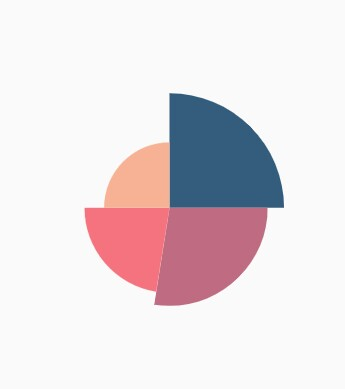# Pie Chart in Flutter Circular Charts (SfCircularChart)

11 Apr 202223 minutes to read

To create a Flutter pie chart quickly, you can check this video.

To render a pie chart, create an instance of `PieSeries`, and add it to the `series` collection property of `SfCircularChart`. The following properties can be used to customize the appearance of pie segment:

``````@override
Widget build(BuildContext context) {
final List<ChartData> chartData = [
ChartData('David', 25),
ChartData('Steve', 38),
ChartData('Jack', 34),
ChartData('Others', 52)
];
return Scaffold(
body: Center(
child: Container(
child: SfCircularChart(
series: <CircularSeries>[
// Render pie chart
PieSeries<ChartData, String>(
dataSource: chartData,
pointColorMapper:(ChartData data,  _) => data.color,
xValueMapper: (ChartData data, _) => data.x,
yValueMapper: (ChartData data, _) => data.y
)
]
)
)
)
);
}

class ChartData {
ChartData(this.x, this.y, [this.color]);
final String x;
final double y;
final Color color;
}``````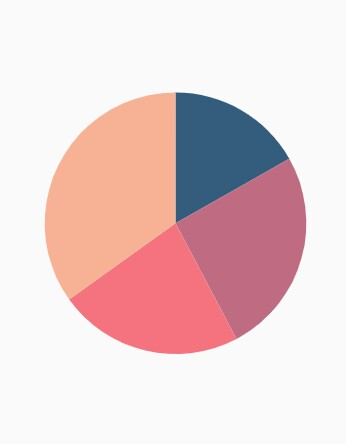## Changing pie size

You can use the `radius` property to change the diameter of the pie chart with respect to the plot area. The default value is `80%`.

``````@override
Widget build(BuildContext context) {
return Scaffold(
body: Center(
child: Container(
child: SfCircularChart(
series: <CircularSeries>[
PieSeries<ChartData, String>(
dataSource: chartData,
xValueMapper: (ChartData data, _) => data.x,
yValueMapper: (ChartData data, _) => data.y,
)
]
)
)
)
);
}``````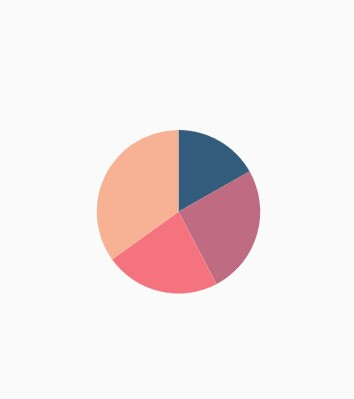## Exploding a segment

You can explode a pie segment by enabling the `explode` property. The following properties can be used to customize the explode options:

``````@override
Widget build(BuildContext context) {
return Scaffold(
body: Center(
child: Container(
child: SfCircularChart(
series: <CircularSeries>[
PieSeries<ChartData, String>(
dataSource: chartData,
xValueMapper: (ChartData data, _) => data.x,
yValueMapper: (ChartData data, _) => data.y,
// Segments will explode on tap
explode: true,
// First segment will be exploded on initial rendering
explodeIndex: 1
)
]
)
)
)
);
}``````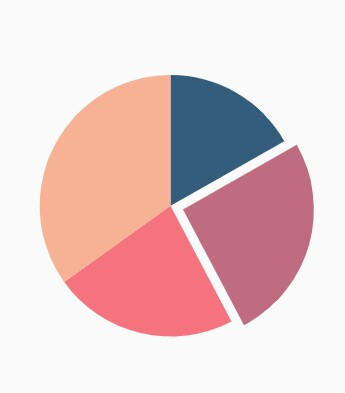## Exploding all the segments

Using the `explodeAll` property of `PieSeries`, you can explode all the pie segments.

``````@override
Widget build(BuildContext context) {
return Scaffold(
body: Center(
child: Container(
child: SfCircularChart(
series: <CircularSeries>[
PieSeries<ChartData, String>(
dataSource: chartData,
xValueMapper: (ChartData data, _) => data.x,
yValueMapper: (ChartData data, _) => data.y,
explode: true,
// All the segments will be exploded
explodeAll: true
)
]
)
)
)
);
}``````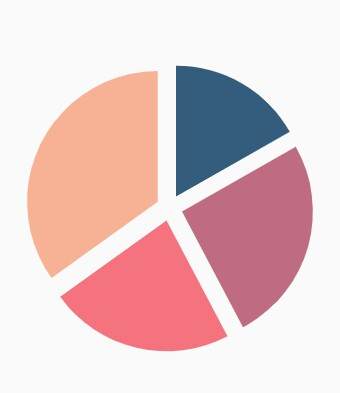## Angle of pie

`SfCircularChart` allows you to render all the data points or segments in semi-pie, quarter-pie, or in any sector using the `startAngle` and `endAngle` properties.

``````@override
Widget build(BuildContext context) {
return Scaffold(
body: Center(
child: Container(
child: SfCircularChart(
series: <CircularSeries>[
PieSeries<ChartData, String>(
dataSource: chartData,
xValueMapper: (ChartData data, _) => data.x,
yValueMapper: (ChartData data, _) => data.y,
// starting angle of pie
startAngle: 270,
// ending angle of pie
endAngle: 90
)
]
)
)
)
);
}``````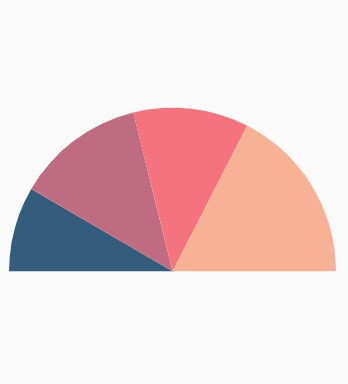## Grouping data points

The small segments in the pie chart can be grouped into others category using the `groupTo` and `groupMode` properties of `PieSeries`. The `groupMode` property specifies the grouping type based on the actual data point value or by points length, and the `groupTo` property sets the limit to group data points into a single slice. The grouped segment is labeled as Others in legend and toggled as any other segment. The default value of the `groupTo` property is null, and the default value of `groupMode` property is `CircularChartGroupMode.point`.

``````@override
Widget build(BuildContext context) {
return Scaffold(
body: Center(
child: Container(
child: SfCircularChart(
series: <CircularSeries>[
PieSeries<ChartData, String>(
dataSource: chartData,
xValueMapper: (ChartData data, _) => data.x,
yValueMapper: (ChartData data, _) => data.y,
groupMode: CircularChartGroupMode.point,
// As the grouping mode is point, 2 points will be grouped
groupTo: 2
)
]
)
)
)
);
}``````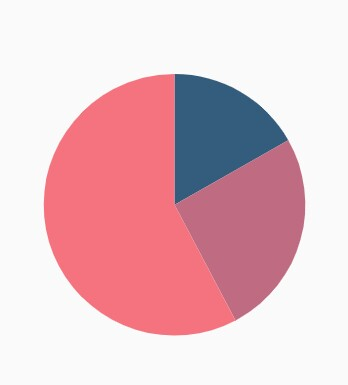## Various radius for each slice

The `pointRadiusMapper` maps the field name, which will be considered for calculating the radius of the data points.

``````@override
Widget build(BuildContext context) {
final List<ChartData> chartData = [
ChartData('USA', 10, '70%'),
ChartData('China', 11, '60%'),
ChartData('Russia', 9, '52%'),
ChartData('Germany', 10, '40%')
];
return Scaffold(
body: Center(
child: Container(
child: SfCircularChart(
series: <CircularSeries>[
PieSeries<ChartData, String>(
dataSource: chartData,
xValueMapper: (ChartData data, _) => data.x,
yValueMapper: (ChartData data, _) => data.y,
// Radius for each segment from data source
pointRadiusMapper: (ChartData data, _) => data.size
)
]
)
)
)
);
}

class ChartData {
ChartData(this.x, this.y, this.size);
final String x;
final double y;
final String size;
}``````